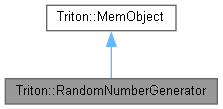Triton Visual simulation library for ocean rendering.
Triton::RandomNumberGenerator Class Referenceabstract

An interface for generating random numbers in Triton. More...

`#include <RandomNumberGenerator.h>`

Inheritance diagram for Triton::RandomNumberGenerator:[legend]
Collaboration diagram for Triton::RandomNumberGenerator:[legend]

## Public Member Functions

virtual double TRITONAPI GetRandomDouble (double start, double end) const =0
Return an evenly distributed random double-precision number within a given range. More...

virtual int TRITONAPI GetRandomInt (int start, int end) const =0
Return an evenly distributed random integer within a given range. More...

virtual void TRITONAPI SetRandomSeed (unsigned int seed)=0
Seeds the random number generator with a given value, to ensure consistent results. More...

## Detailed Description

An interface for generating random numbers in Triton.

Subclass this interface and pass an instance to Environment::SetRandomNumberGenerator in order to override Triton's default usage of rand(). This may be useful for enforcing deterministic behavior across several channels.

## ◆ GetRandomDouble()

 virtual double TRITONAPI Triton::RandomNumberGenerator::GetRandomDouble ( double start, double end ) const
pure virtual

Return an evenly distributed random double-precision number within a given range.

Parameters
 start The lowest value in the range end The highest value in the range
Returns
An evenly distributed random number within the range.

## ◆ GetRandomInt()

 virtual int TRITONAPI Triton::RandomNumberGenerator::GetRandomInt ( int start, int end ) const
pure virtual

Return an evenly distributed random integer within a given range.

Parameters
 start The lowest value in the range end The highest value in the range
Returns
An evenly distributed random number within the range.

## ◆ SetRandomSeed()

 virtual void TRITONAPI Triton::RandomNumberGenerator::SetRandomSeed ( unsigned int seed )
pure virtual

Seeds the random number generator with a given value, to ensure consistent results.

Parameters
 seed A value used to seed the random number generator's sequence of psuedo-random numbers.

The documentation for this class was generated from the following file: